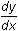Module 13 - Implicit Differentiation Introduction | Lesson 1 | Lesson 2 | Self-Test Introduction In this module you will study expressions where y is not explicitly defined as a function of x. In these expressions the relation between y and x is implicit, i.e., implied, and they are called implicit relations. It is often difficult and sometimes impossible to solve for y in terms of x. Therefore, different methods are needed to graph an implicit relation on the TI-89 and to find its derivative. Lesson Index: 13.1 - Graphs of Implicit Relations 13.2 - Implicit Differentiation After completing this module, you should be able to do the following: Use the TI-89 to graph an implicit relation Findby hand using implicit differentiation Use the TI-89 to findfor implicit relations Find tangent lines to implicitly defined curves Use the TI-89 to solve nonlinear systems of equations Next > ©Copyright 2007 All rights reserved. | Trademarks | Privacy Policy | Link Policy